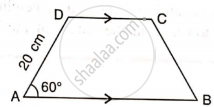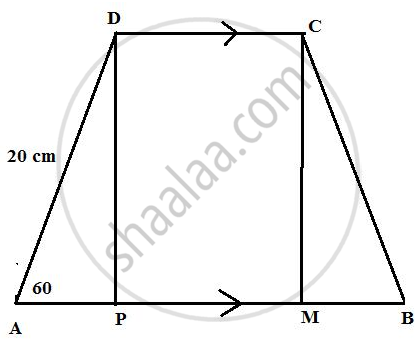# In Trapezium Abcd, as Shown, Ab // Dc, Ad = Dc = Bc = 20 Cm and a = 60°. Find: Length of Ab - Mathematics

Sum

In trapezium ABCD, as shown, AB // DC, AD = DC = BC = 20 cm and ∠ A = 60°. Find: length of AB#### Solution

First, draw two perpendiculars to AB from point D and C respectively. Since AB || CD therefore PMCD will be a rectangle.
Consider the figure,From right triangle ADP we have

cos 60° = "AP"/"AD"

(1)/(2) = "AP"/(20)

AP = 10

Similarly from the right triangle BMC we have BM = 10 cm.

Now from the rectangle PMCD we have CD = PM = 20 cm.

Therefore,
AB = AP + PM + MB = 10 + 20 + 10 = 40 cm.

Concept: Solution of Right Triangles
Is there an error in this question or solution?

#### APPEARS IN

Selina Concise Mathematics Class 9 ICSE
Chapter 24 Solution of Right Triangles [Simple 2-D Problems Involving One Right-angled Triangle]
Exercise 24 | Q 8.1 | Page 303